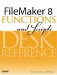# Evaluate()

Category: Logical

 Syntax: Evaluate ( expression {; [ field1; field2; ...]} )

Parameters:

expressionAny valid calculation formula, field containing a valid formula, or expression returning a valid formula.

field(n)A list of optional fields that can then serve to trigger a re-evaluation; the expression re-evaluates when any of the included fields are updated.

Parameters in curly braces { } are optional. The optional field list must be enclosed by square brackets when there are multiple parameters.

Data type returned: Text, Number, Date, Time, Timestamp, Container

Description:

The Evaluate() function returns the results obtained by evaluating expression.

The optional second parameter is a list of fields on which the calculation becomes dependent. When any of those fields are modified, the Evaluate() function re-evaluates the expression specified by the first parameter.

The Evaluate() function expects that the first parameter passed to it is a string that contains a formula of some sort. If you are passing a literal string, as in the fourth of the following examples, using the Quote() function ensures that any quotation marks in the formula itself are properly encoded. If the first parameter is a field name or an expression, that field or expression is expected to return a formula, which the Evaluate() function then evaluates. In a nutshell, if the first parameter is not surrounded by quotation marks, the result of whatever field or expression is provided is evaluated.

Note that the execution of the expression does occurin other words, do not think of Evaluate() as a "testing function" making use of its own memory space. If your expression modifies a global or local variable (using the Let() function), any applicable changes will be applied. If you need a calculation "scratch pad," consider using the Evaluate Now function of the FileMaker Advanced Data Viewerthough there again, any "side-effect" modifications of variables will occur "for real," a good example of why modifying variables from within calculations can be a questionable practice.

Examples:

Function

Results

Evaluate(MyFormula)

Returns 8 if MyFormula contains the string 5+3.

Evaluate(MyFormula)

Returns 4 if MyFormula contains the string Length (FirstName) and FirstName contains Fred.

Evaluate("MyFormula")

Returns a text string, "5+3" if MyFormula contains the string 5+3.

Evaluate(Quote ("The comment field was last updated on " & Get (CurrentDate) & " by " & Get (AccountName)); CommentField)FileMaker 8 Functions and Scripts Desk Reference
ISBN: 0789735113
EAN: 2147483647
Year: 2004
Pages: 352

Similar book on Amazon